Recent results

(1) A novel equation to precisely predict the conductivity of the ternary composites  (Composites Science and Technology, 150, 24-31.)

The binary CPC system with only one carbon filler can be detailed described already, however, to predict the conductivity of the ternary CPC system was always a challenge due to the interaction between two conductive fillers. In our recent work, a parameter was proposed to describe this interaction as a synergy, and the complete equation can be used to the precisely predict the conductivity of the ternary composites PMMA/CB/CF. Fig. 1 (A) presents logarithm value of conductivity of composite filament vs filler volume fraction of CFs and CB in a waterfall plot diagram. Fig. 1 (B) shows experimental data as filled symbols while the simulation curves with predicted data represented by solid lines.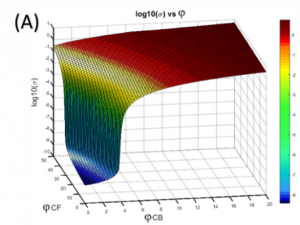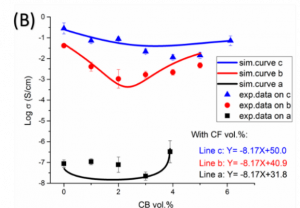Fig. 1 (A) Logarithm value of conductivity of composite filament vs. filler volume fraction of CFs and CB in a waterfall plot diagram (B) Logarithm value of conductivity of composite filament vs. filler volume fraction of CB in a 2-D coordinates system. Filled symbols represent experimental data while solid lines represent the simulation curves with predicted data, where line a-c are chosen to present the volume fraction ratio between CB and CFs.

(2) A Novel definition on synergistic effect between CB and CNTs in a ternary CPC system (Nanotechnology, 2019, 30)

In a recent work from LSP, the ternary PMMA/CB/CNTs composites filament was produced (Fig. 2 (A)). A success validation for binary CPC system is presented in Fig. 2 (B). The 4th synergy and the comparison between the experimental data and the predicted curve are presented in Fig. 2 (C). All the available literature on the synergistic effect between CB and CNTs in a ternary CPC system has been carefully discussed, and a quantifiable “synergasm” between CNTs and CB is defined as the novel 5th synergistic effect in this work.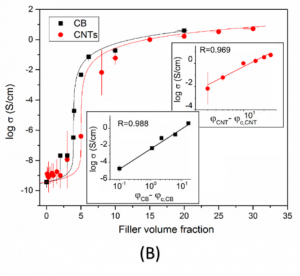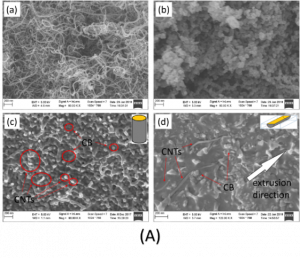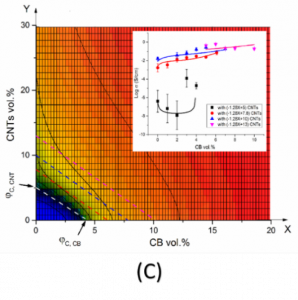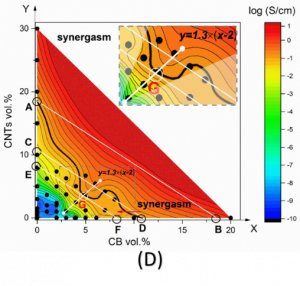Fig. 2 (A) Morphology of the Nanoparticles (a) CNTs and (b) CB used in this study; Morphology of the anisotropic ternary CPCs filament with 5.44 vol. % CNTs and 6 vol. % CB using SEM: (c) the cross-section of the specimen; (d) the cross-section of the specimen along the fiber axis. (B) The logarithm of the conductivity vs. filler volume fraction of CNTs and CB, respectively. The solid lines show the best fit with the GEM equation. (C) Logarithm of the composite conductivity versus volume filler fraction of CNTs and CB in a contour plot. The inset shows experimental- and modelled data along the four dotted lines. (D) The logarithm of the composite conductivity vs volume filler fraction of CNTs and CB in a contour plot diagram, defining a quantifiable “synergasm” between CNTs and CB. The inset synergism map illustrates the synergistic effect of the different compositions of CNTs and CB from this study.